## research papersBIOLOGICALCRYSTALLOGRAPHY
ISSN: 1399-0047
Volume 55| Part 11| November 1999| Pages 1872-1877

## Evaluation of macromolecular electron-density map quality using the correlation of local r.m.s. density

aStructural Biology Group, Mail Stop M888, Los Alamos National Laboratory, Los Alamos, NM 87545, USA, and bBiophysics Group, Mail Stop D454, Los Alamos National Laboratory, Los Alamos, NM 87545, USA
*Correspondence e-mail: terwilliger@lanl.gov

(Received 28 December 1998; accepted 27 July 1999)

It has recently been shown that the standard deviation of local r.m.s. electron density is a good indicator of the presence of distinct regions of solvent and protein in macromolecular electron-density maps [Terwilliger & Berendzen (1999). Acta Cryst. D55, 501–505]. Here, it is demonstrated that a complementary measure, the correlation of local r.m.s. density in adjacent regions on the unit cell, is also a good measure of the presence of distinct solvent and protein regions. The correlation of local r.m.s. density is essentially a measure of how contiguous the solvent (and protein) regions are in the electron-density map. This statistic can be calculated in real space or in reciprocal space and has potential uses in evaluation of heavy-atom solutions in the MIR and MAD methods as well as for evaluation of trial phase sets in ab initio phasing procedures.

### 1. Introduction

The field of macromolecular crystallography is rapidly moving towards the automation of many aspects of structure determination. Processing of diffraction images is now routine and nearly automatic (Otwinowski & Minor, 1997; Leslie, 1993). Identification of heavy-atom sites in MIR and MAD data sets can often be performed in a hightly automated fashion even in cases where many sites are present (Terwilliger & Berendzen, 1999a; Terwilliger et al., 1987; Chang & Lewis, 1994; Vagin & Teplyakov, 1998; Sheldrick, 1990; Miller et al., 1994; Brunger et al., 1998) and an automated procedure has recently been developed that can carry out all aspects of scaling, heavy-atom location, refinement and phase calculation (Terwilliger & Berendzen, 1999b). For macromolecular crystals that diffract to very high resolution, procedures based on combinations of real-space and reciprocal-space direct methods have been used to determine phases without MIR or MAD experimental data with considerable success (e.g., Deacon et al., 1998; Ealick, 1997). Model building of macromolecules into electron-density maps is also being automated (e.g. Perrakis et al., 1997; Zou & Jones, 1996).

With the automation of structure solution, reliable methods for evaluating the quality of electron-density maps are becoming increasingly important. In the MIR and MAD methods, for example, the main criterion for judging the quality of phasing is simply the interpretability of the resulting electron-density map. This works well when an experienced crystallographer is evaluating a map, but is not as useful in the context of automated structure determination. Even more importantly, when direct methods are used to solve protein structures, many phase sets need to be evaluated before a correct one is identified. The choice of an optimal `figure of merit' for evaluating the relative qualities of these phase sets is of major importance (Deacon et al., 1998).

There are several characteristics of macromolecular electron-density maps which are particularly well suited for use as measures of quality. These include the connectivity of electron density corresponding to polypeptide chains in protein-crystal maps (Baker et al., 1993), the presence of distinct regions of protein and solvent (Wang, 1985; Xiang et al., 1993; Podjarny et al., 1987; Abrahams et al., 1994; Zhang & Main, 1990) and histogram matching of electron densities (Zhang & Main, 1990; Goldstein & Zhang, 1998). Several procedures for automatic evaluation of the quality of electron-density maps have recently been described. Most of these are real-space procedures, but one can be calculated in reciprocal space. One real-space procedure is based on the connectivity of the electron-density map (Baker et al., 1993). The measure of quality is essentially the number of connected segments that can be identified in the map. Another real-space procedure is based on the non-random distribution of electron densities in the unit cell (Goldstein & Zhang, 1998). Histogram-matching techniques are used to compare the distributions in a trial map with those expected of macromolecules containing distinct regions of solvent and macromolecule and thereby to evaluate the quality of the trial map.

A third procedure for evaluating map quality, which can be carried out in either real space or reciprocal space, is based, like the histogram-matching procedure, on the distinction between protein and solvent regions (Terwilliger & Berendzen, 1999a). The regions in a protein crystal that contain disordered solvent are relatively featureless. Consequently, those regions have a low local variation of electron density. In contrast, regions containing the macromolecule have atoms at some positions and not at others, leading to a high local variation of electron density. The presence of regions of both low local variation and high local variation can be detected by calculating the standard deviation over the asymmetric unit of local r.m.s. electron density (Terwilliger & Berendzen, 1999a; Terwilliger, 1999). This standard deviation is high when the electron-density map has well defined protein and solvent regions and is low for maps calculated with random phases.

Although the standard deviation of local r.m.s. electron density and the histogram-matching approaches are useful in evaluating whether distinct regions of protein and solvent exist in a map, they do not take full advantage of the spatial extent and separation of protein and solvent regions. The standard deviation, for example, is only a measure of how much variation there is of local r.m.s. electron density from place to place in the unit cell. It cannot distinguish between cases where regions of low and high local r.m.s. electron density are very small and are interspersed among each other, and the very different case where the regions of low and high local r.m.s. electron density are contiguous and very large in extent. Correct macromolecular electron-density maps ordinarily correspond to the second case, where regions of high and low r.m.s. electron density are each very large and contiguous. The extents of protein and solvent regions are often so large that there are only one or a few distinct regions of protein and of solvent in the asymmetric unit.

Here, we present a measure of the quality of macromolecular electron-density maps which is based on the spatial separation of large contiguous regions of high or low r.m.s. electron density. This new measure is complementary to the standard deviation of local r.m.s. electron density we have previously used and can be combined with it to generate a composite measure of quality which is more useful in discriminating correct from incorrect maps than either measure alone. The measure does not depend on atomicity and can therefore be used with X-ray data at resolutions as low as 4 Å. We show that it can be calculated in either real or reciprocal space.

### 2. Methods

#### 2.1. Calculation of the correlation of local r.m.s. density from an electron-density map

The correlation of local r.m.s. electron density in neighboring regions of the unit cell was obtained from electron-density maps calculated on a grid with a spacing of approximately one-third of the resolution of the data, without including the F000 term in the Fourier synthesis. To calculate the correlation of the local r.m.s. density, the asymmetric unit of the map is divided into cubes with edges of 5 grid units. (The method is relatively insensitive to the size of the cubes over the edge range 3–9 units for maps calculated at a resolution of 3 Å.) Partial cubes with less than half the volume of a full cube are ignored. The r.m.s. electron density in each cube is calculated using the grid points in the cube which are contained within the asymmetric unit of the crystal. The correlation coefficient for r.m.s. electron density is then calculated for all pairs of neighboring cubes.

#### 2.2. Reciprocal-space calculation of correlation of local r.m.s. density

A means of calculating the correlation of the local r.m.s. density in reciprocal space would be useful in applications such as evaluation of phase sets in ab initio methods for phase determination. If a reciprocal-space calculation were used, then fewer Fourier transforms would have to be calculated. We have therefore developed a reciprocal-space formulation of this measure of map quality. To do this, we have used an approach similar to the one we recently described for calculation of σR, the standard deviation of local r.m.s. density of a map (Terwilliger, 1999).

Because the procedure for calculating the correlation of local r.m.s. density described above is not well suited to a reciprocal-space description, we first reformulated this calculation slightly, substituting local mean-square density for local r.m.s. density so as not to require a square-root calculation. As these two quantities are very closely related, we anticipated that the two calculations would yield very similar results.

The calculation of correlation of local mean-square density is based on the local mean-square density of the map,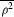(x), which we will define here to be averaged over a region defined by a Gaussian function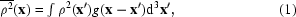where g(x) is a three-dimensional Gaussian function with unit volume and a variance (in each direction x, y, z) of σ2,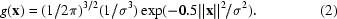The goal is to calculate a quantity for a map that describes how correlated the local mean-square density(x) at coordinates x is with the local mean-square density(x + x′) a distancexaway at coordinates x + x′. This correlation CC is calculated over the entire unit cell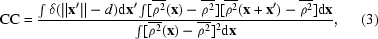where δ(xd) is a three-dimensional Dirac distribution (zero unlessx= d) and is normalized so that it has unit volume;is the mean-square density in the map.

(3) can be used to calculate the correlation of local mean-square density in a map in real space. To calculate the same quantity in reciprocal space, we first rewrite it as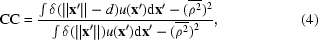where the correlation u(x′) between local mean-square densities separated by the vector x′ is given by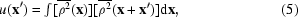which can be recognized as the Patterson function of the local mean-square density(x).

Next, we follow our previous approach (Terwilliger, 1999) and note that the coefficients Bh of the Fourier series representation of ρ2(x) can be calculated from the structure factors Fh using the relation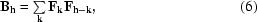summing over all values of k. The values of Fk are the same as those used to calculate an electron-density map [ρ(x)]. We now take advantage of the fact that the local mean-square density(x) in (1) is the convolution of ρ2(x) with the Gaussian function g(x). The coefficients Rh of the Fourier series representation of the convolution(x) are then simply the products of the coefficients Bh and the coefficients Gh for the Fourier series representation of the Gaussian,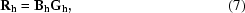where the coefficients of the Fourier transform of the Gaussian function are given by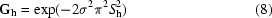and Sh is the magnitude of the scattering vectorh= 2sinθ/λ.

Since u(x′) (5) is the Patterson function of(x), the coefficients Uh in its Fourier transform are the squares of the magnitudes of Rh (7),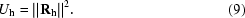The final set of coeffficients needed (Th) are those for δ(x− d), an infinitely thin shell of radius d with unit volume. These can be shown to be given byWe are now in a position to evaluate (4) in reciprocal space. The numerator of (4) contains two terms, the integral of the product δ(xd)u(x′) and the square of the mean value of ρ2. Using the fact that the integral over the unit cell of any term in a Fourier series with any other term is zero unless the terms have identical indices and noting that both δ and u are real functions, the integral of the product can be reduced to the expression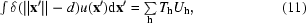where the sum is over all indices h. Similarly, the square of the mean value of ρ2 can be rewritten using only h = 000 terms as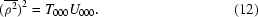The denominator in (4) is identical to the numerator, except that the separation d is zero in the denominator, yielding the result that Th = 1 for all indices h. Substituting using (9), this yields the following reciprocal-space expression for the correlation of local mean-square density,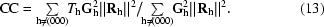All of the quantities in (13) are readily calculated using (7), based on the same amplitudes and phases of structure factors (Fh) which would be used to calculate an electron-density map and using the expressions for Gh and Th in (8) and (10), respectively.

(13) has a quite simple interpretation. The numerator is the average value at a radius d of the Patterson function of the squared electron density after smoothing. The Th terms represent the selection of the distance d. The Gh terms represent the Gaussian smoothing (averaging) of the Patterson function and the Rh are the coefficients of the Fourier series for the squared electron density. Another way to say this is that the numerator of (13) is the correlation of the squared electron density, after smoothing, at a distance d. The denominator is the value of the same Patterson function at the origin. The denominator is the correlation of the squared electron density, after smoothing, with itself. The overall CC is the ratio of these two quantities.

Two parameters are required to evaluate (13), the variance σ2 of the Gaussian used to smooth the Patterson function (2) and the radius d at which the correlation is calculated (3). Our analysis of the real-space measure of correlation of local r.m.s. density above showed that the precise size of the region averaged (corresponding roughly to σ in the reciprocal-space version) had only a small effect in the range 3–9 Å. We chose the width of the Gaussian distribution σ to be 3 Å so that the local regions to be compared were largely contained within a region of dimensions 5 Å. We then chose the separation d to be twice this so that the compared regions would not overlap significantly.

### 3. Results and discussion

We used model data to examine the utility of the correlation of local r.m.s. electron density in adjacent regions of a map in distinguishing between electron-density maps of high and low quality. Model structure factors were generated using ­coordinates determined recently in our laboratory of a de­halogenase enzyme from Rhodococcus species ATCC 55388 (American Type Culture Collection, 1992), which contained 316 amino-acid residues and crystallized in space group P21212 with unit-cell dimensions a = 94, b = 80, c = 43 Å (J. Newman, personal communication). The resolution range used in the model calculations was 3–20 Å. Varying phase errors were then applied to these model structure factors to yield 4830 phase sets with mean values of the effective figure of merit 〈cosΔφ〉 ranging from 0.0 to 1.0 (Δφ is the phase error).

Two automated measures of the quality of each electron density were then calculated for each map and compared with the true effective figure of merit of the map (obtained using the known phase errors). The two measures were the standard deviation of local r.m.s. electron density (SD; Terwilliger & Berendzen, 1999a) and the correlation of local r.m.s. electron density (CC) described here. Fig. 1shows the values of each measure of map quality for the 4830 phase sets we examined. The two criteria have similar overall characteristics. For maps based on phase sets with effective figures of merit greater than about 0.4, each criterion appears to be strongly related to the figure of merit of the map. For maps of lower quality, the two criteria are weakly related to the figure of merit of the map.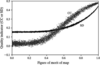Figure 1 Standard deviation of local r.m.s. density (SD) and correlation of local r.m.s. density (CC) for model data sets. The values of SD and CC were calculated for 4830 model phase sets as described in the text. The figure of merit of each map is the value of 〈cosΔφ〉 for that map. The values of SD (circles) and CC (squares) are shown for each phase set.

The utility of each criterion for ranking maps in order of quality is examined in more detail in Fig. 2(a). All pairs of phase sets which differed in figure of merit by 0.05 ± 0.025 were listed. For each pair, it was then determined whether the standard deviation of local r.m.s. density (SD) or correlation of local r.m.s. density (CC) criteria would have correctly identified the better of the two phase sets. The fraction of correct decisions of this type are plotted in Fig. 2(a) as a function of map quality (figure of merit). For pairs of maps with effective figure of merit of less than 0.2, neither criterion is very useful in identifying the better of the two phase sets. For pairs of maps with figures of merit from 0.2 to 0.4, however, Fig. 2(a) illustrates that the new correlation criterion (CC) is more likely to identify the better of the two phase sets than the standard-deviation criterion (SD). For example, the likelihood that the SD criterion would correctly identify the better of two maps with an average effective figure of merit of 0.22 and differing by 0.05 is about 0.52, while the CC criterion would have a likelihood of 0.56. For maps with an effective figure of merit above about 0.5, both criteria are very reliable, but the SD criterion is more useful than the correlation CC.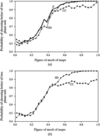Figure 2 Probability of identifying the better of two model phase sets. (a) All pairs of phase sets in Fig. 1differing in figure of merit by 0.05 ± 0.025 were examined. The fraction of cases in which the SD or CC values were higher for the phase set with the higher figure of merit is plotted as a function of the mean figure of merit for the two maps. (b) As in (a), except that a different set of 4000 model phase sets were used and the analysis was performed in reciprocal space. The 364 terms in the series representations of SD or CC (see text) with the smallest values of Gh were included. The width (standard deviation) of the Gaussian function used to define the local region was σ = 3 Å and the radius of the shell function for the calculation of CC was 10 Å.

A composite criterion Z based on both the SD and CC measures of map quality was also tested. This composite was calculated as the sum of the SD and CC measures, after normalizing each based on their means and standard deviations for the data points in Fig. 2(a) in the range of map quality 0.0–0.1. This normalization procedure is a simple way of weighting the two criteria so that equal changes in each criterion relative to their respective standard deviations lead to equal changes in Z. Fig. 2(a) shows that the composite score Z is more useful than either of the individual criteria in identifying the better of two phase sets. In the range of map quality 0.2–0.4, the composite Z is slightly better than the correlation (CC) criterion and much better than the SD criterion. In the range 0.4–0.5, it is much better than either the SD or CC criteria, and for maps with quality above 0.5, the composite Z is about equal to the SD criterion and much better than the correlation CC.

Both of the criteria examined here (SD and CC) can be calculated in either real space or reciprocal space. Fig. 2(b) shows the results of a test with 4000 model phase sets, where SD and CC were calculated in reciprocal space, as described in previous work (Terwilliger, 1999), or with (13), respectively. The reciprocal-space calculations are carried out with a series representation (13) in which the Gaussian terms Gh strongly reduce the contribution of high-order terms. Consequently, we only used the lowest order terms with values of Gh > 0.1 in the series for these calculations. As anticipated, the reciprocal-space calculations yielded measures of both SD and CC which have properties very similar to those calculated for related quantities in real space.

Model data sets were also used to test the range of resolution over which the correlation of local r.m.s. density (CC) was a useful measure of map quality. Fig. 3is a repetition of the CC analysis in Fig. 2(a) for maps calculated at three resolutions: 3, 4 and 6 Å. Fig. 3shows that the utility of the correlation CC in distinguishing between maps of slightly different quality is best at higher resolution, but is still of some use for maps calculated at a resolution as low as 6 Å.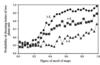Figure 3 Effect of the resolution of the map on the probability of identifying the better of two phase sets.

The correlation of local r.m.s. density (CC) was tested for utility with real data by including it in a repetition of the automated structure determination (Terwilliger & Berendzen, 1999b) of the Rhodococcus dehalogenase based on experimental data (J. Newman, unpublished data) at a resolution of 2.8 Å. As the structure of the dehalogenase has been refined at a resolution of 1.5 Å, the quality of electron-density maps calculated from each trial heavy-atom solution during the structure determination could be assessed using the correlation coefficient to the model map (Fig. 4). Anomalous differences were not used in this test, so heavy-atom solutions were translated and inverted as necessary to match the origin used for the model structure. Fig. 4shows the relationship between the quality of electron-density maps calculated during this automated dehalogenase structure determination and the values of the standard deviation SD (Fig. 4a) and correlation CC (Fig. 4b) of local r.m.s. density. The linear correlation coefficient for the data in Fig. 4(a) (SD) is 0.89; for CC it is 0.90. We conclude that both criteria would be very useful in ranking trial electron-density maps.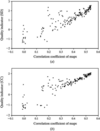Figure 4 SD and CC of maps calculated during a structure determination with real data. Automated structure determination of a dehalogenase enzyme was carried out using SOLVE (Terwilliger & Berendzen, 1999b), as described in the text. The 178 trial heavy-atom solutions examined during the structure determination were each used to calculate an electron-density map. The values of SD (a) and CC (b) calculated from these maps are plotted as functions of the correlation of the map to a map calculated with phases based on a refined model of the dehalogenase.

### 4. Conclusions

The standard deviation and correlation of local r.m.s. electron density in a map are complementary properties of the map. Each statistic can be a good indicator of the quality of macromolecular electron-density maps. The standard deviation of local r.m.s. density is essentially a measure of how much variation there is in the local roughness of the map from place to place in the map. The correlation of local r.m.s. density, in contrast, is a measure of how contiguous the flat (or rough) regions of the map are. A high-quality map of a macromolecular structure with significant solvent regions will have both a high standard deviation and a high correlation of local r.m.s. electron density. Our results from model and real data indicate that both statistics are useful and that a combination of the two statistics is more useful than either alone in ranking the quality of electron-density maps.

We have recently shown that the standard deviation of local r.m.s. density can be expressed in a reciprocal-space formulation (σR; Terwilliger, 1999). The reciprocal-space formulation can be calculated rapidly using a relatively small number of terms in a series approximation. It can also be differentiated and therefore potentially used as a target for optimizing phases. A similar approach has been applied here to express the correlation of local r.m.s. density in reciprocal space. These real-space and reciprocal-space formulations have potential applications in ranking phase sets obtained from heavy-atom solutions to MIR and MAD experiments as well as in density-modification and direct-methods approaches to macromolecular phase determination.

### Acknowledgements

The authors are grateful for support from the National Institutes of Health and the US Department of Energy, and would like to thank J. Newman for the use of the dehalogenase data.

### References

This is an open-access article distributed under the terms of the Creative Commons Attribution (CC-BY) Licence, which permits unrestricted use, distribution, and reproduction in any medium, provided the original authors and source are cited.BIOLOGICALCRYSTALLOGRAPHY
ISSN: 1399-0047
Volume 55| Part 11| November 1999| Pages 1872-1877